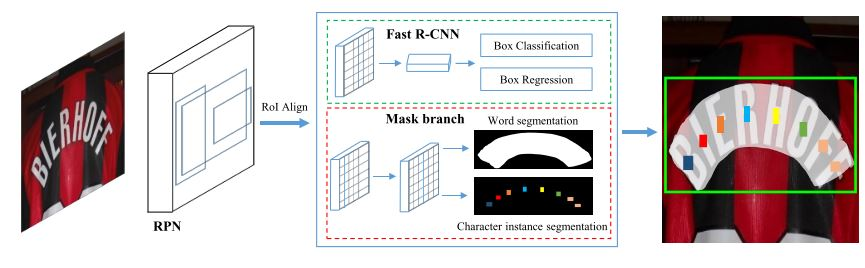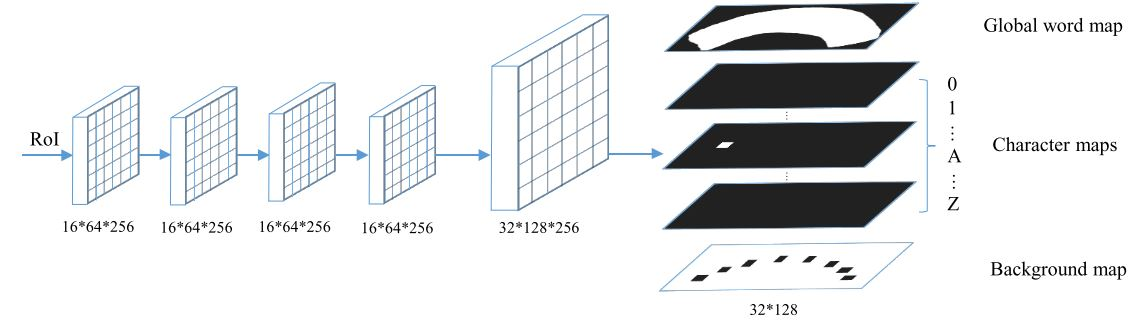0%

### ArchitectureFigure 1. Model architecture

• Backbone：FPN + ResNet50，用来提取特征图。
• RPN：生成 text proposals，然后使用 RoI Align 提取 RoI 特征。
• Fast RCNN：对 text proposals 进行更精确地分类和回归。
• Mask Branch：包含 text instance 分割和 character 分割任务。如 figure 2 所示，会输出一个 global word map，36 个 character maps 和一个 background map。character 的 Bbox 被缩小成原来的四分之一。

### Loss Function

$L = L_{rpn} + \alpha_1 L_{rcnn} + \alpha_2 L_{mask} \\\\ L_{mask}=L_{global}+\beta L_{char}$

$L_{global}= - \frac{1}{N} \sum_{n=1}^{N} [y_n \times \log(S(x_n))+(1-y_n) \times \log(1-S(x_n))]$

$L_{char}$ 是一个加权的 soft-max 损失， $Y$$X$ 对应的 ground truth：

$L_{char}= - \frac{1}{N} \sum_{n=1}^{N} W_n \sum_{t=0}^{T-1} Y_{n,t} \log(\frac{e^{x_{n,t}}}{\sum_{k=0}^{T-1}e^{x_{n,k}}})$

### Inference

Pixel Voting 流程：

• 将 background map 进行二值化得到所有的字符区域；
• 对于所有的 character maps 求每个字符区域的像素得分均值，把得分最高的字符类别得分设为该区域输出的字符；
• 把所有的字符从左到右连接得到字符序列。

### My Thoughts

• Mask TextSpotter 本质是用 Faster RCNN 进行目标定位，然后使用目标分割来识别字符，那么可以替换分割算法提升效果。
• 之前的 text spotter 模型都是先分开训练，再联合训练，这个能直接联合训练。
• 英文的字母较少，在中文上可能不行。
•  [2018-ECCV] Mask TextSpotter: An End-to-End Trainable Neural Network for Spotting Text with Arbitrary Shapes paper
•  [2017-ICCV] Deep TextSpotter: An End-to-End Trainable Scene Text Localization and Recognition Framework papercode
•  [2017-ICCV] Towards end-to-end text spotting with convolutional recurrent neural networks. paper